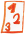Custom math worksheets at your fingertips# Details for problem "Triangle missing value area, side or height"

Quickname: 3335

## Summary

One of the values area, length of a side or corresponding height of a triangle are given. Determine the missing value.

## Example## Description

This problem is about the formula for a triangle's area according to the formula A=c*hc/2. Two of the values involved are given, the third one is to be determined.

Two of these values are given for each problem: The length of the side, the length of the corresponding height and the area. Which of these are given can be configured. The third value has to be calculated.

As a small difficulty, useless values such as additional side length or height specifications can be scattered in. The correct specifications must then be selected for the calculation.

The number of tasks is also selectable.

Download free worksheets for this math problem here. The worksheet contains the problems only, the solutions sheet includes the answers. Just click on the respective link.

•Worksheet 1Solution sheet with answers
•Worksheet 2Solution sheet with answers
•Worksheet 3Solution sheet with answers

If you can not see the solution sheets for download, they may be filtered out by an ad blocker that you may have installed. If this is the case, please allow ads for this page and reload the page. The solution sheets will then reappear.

• Do the sample worksheets do not really fit?
• Do you need more math worksheets, with a different level of difficulty?
• Would you like to combine different problems on a worksheet and adjust them to your needs?
• As a teacher, you can put together your own worksheets using the automatically generated math problems provided.
With a free initial credit, you can start creating your own math worksheets in a few minutes.

It does not cost anything to try! Register here, to create custom worksheets now!

## Customization options for this problem

Parameter
Possible values
Number of problems
1, 2, 3, 4, 5, 6, 7, 8, 9, 10
Given values
any two, side and height, height and area, side and area
Yes, NoDeutsche Version dieser Aufgabe
These informational pages with samples describe math problems that can be combined on custom math worksheets with solutions for home and school use.
Deutsche Seiten
×